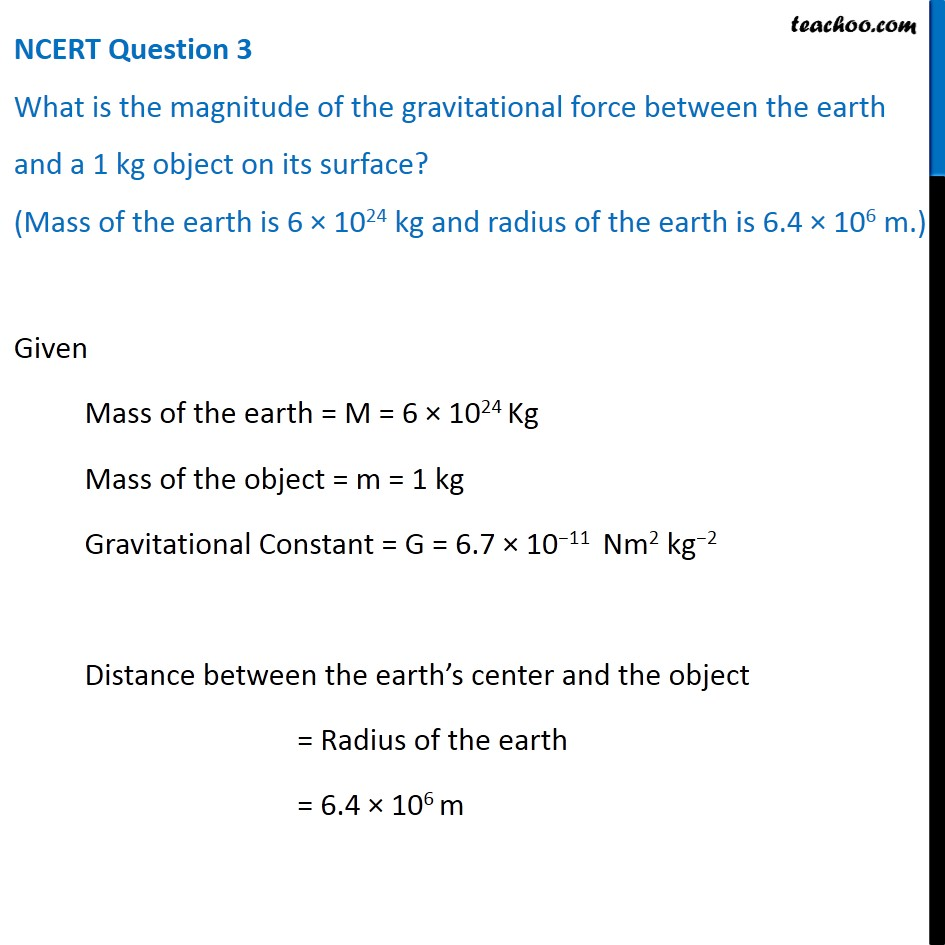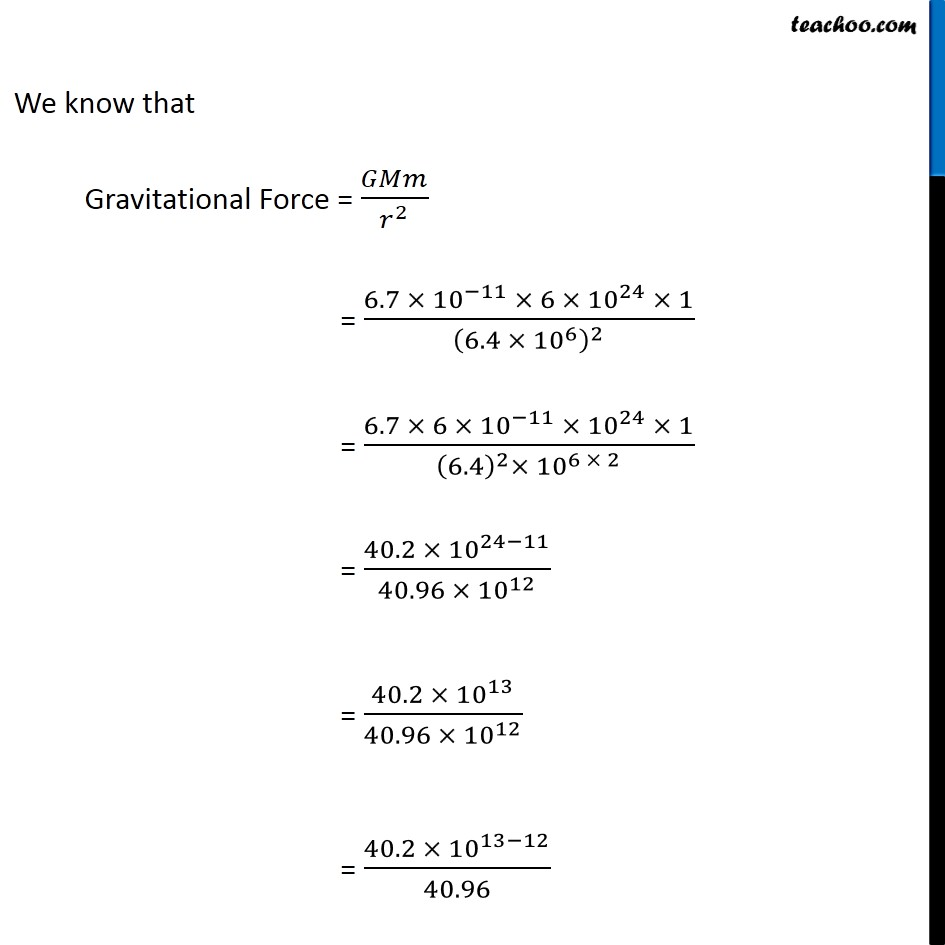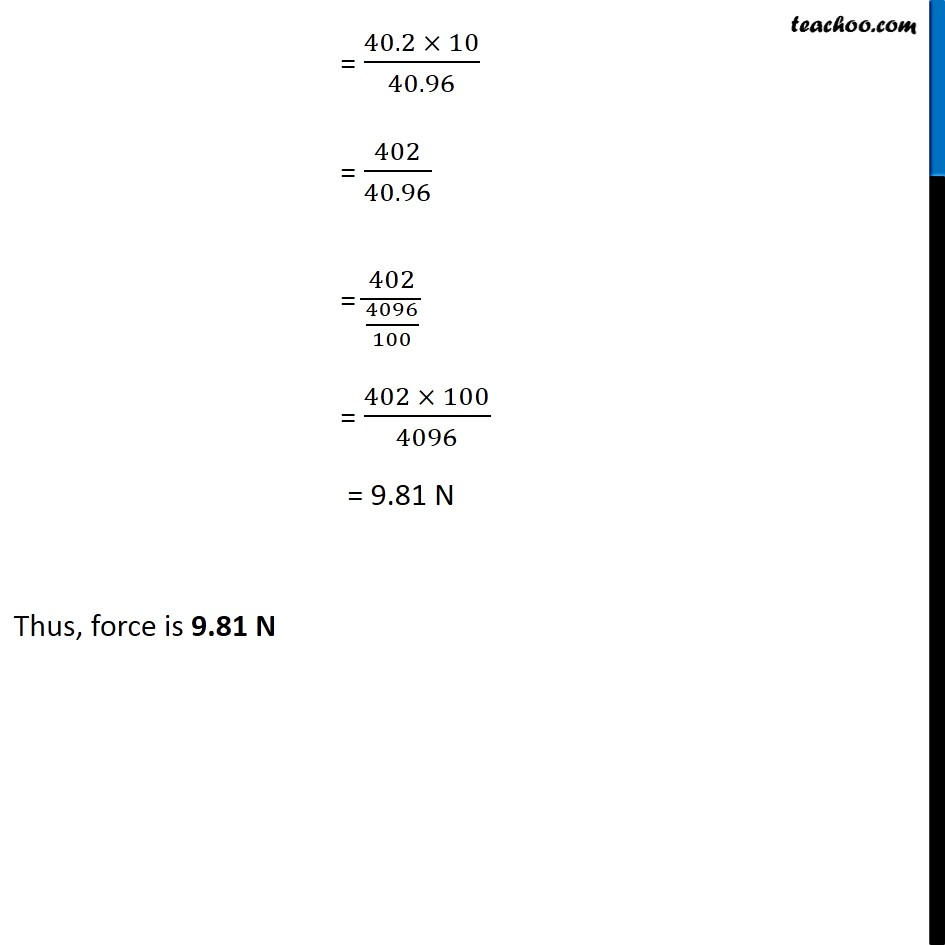NCERT Questions

Class 9
Chapter 10 Class 9 - GravitationLearn in your speed, with individual attention - Teachoo Maths 1-on-1 Class

### Transcript

NCERT Question 3 What is the magnitude of the gravitational force between the earth and a 1 kg object on its surface? (Mass of the earth is 6 × 1024 kg and radius of the earth is 6.4 × 106 m.) Given Mass of the earth = M = 6 × 1024 Kg Mass of the object = m = 1 kg Gravitational Constant = G = 6.7 × 10−11 Nm2 kg−2 Distance between the earth’s center and the object = Radius of the earth = 6.4 × 106 m We know that Gravitational Force = 𝐺𝑀𝑚/𝑟^2 = (6.7 × 〖10〗^(−11) × 6 × 〖10〗^24 × 1)/(6.4 ×〖 10〗^6 )^2 = (6.7 × 6 × 〖10〗^(−11) × 〖10〗^24 × 1)/((6.4)^2×〖 10〗^(6 × 2) ) = (40.2 × 〖10〗^(24−11))/(40.96 ×〖 10〗^12 ) = (40.2 × 〖10〗^13)/(40.96 ×〖 10〗^12 ) = (40.2 × 〖10〗^(13−12))/40.96 = (40.2 × 10)/40.96 = 402/40.96 = 402/(4096/100) = (402 × 100)/4096 = 9.81 N Thus, force is 9.81 N